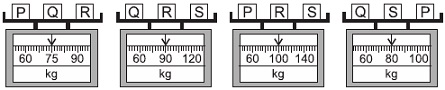# User Forum

Subject :IMO    Class : Class 4

Find the total weight of Q and R.A55 kg
B45 kg
C85 kg
D50 kg

Solution can be much easier when we add all the 4 equation, which gives3 (P Q R S)=345; I.e., P Q R S=115; now subtracting each equation from this we can get the values of p=25; q=15; r=35 and s=40; which means q r=50

Class : Class 4

## Ans 2:

Class : Class 4
Spaces is " ", the value disappeared after I posted the comment

## Ans 3:

Class : Class 4
How is this question done

## Ans 4:

Class : Class 4
There are four equations P Q R=75; Q R S=90; P R S=100; Q S P=80Add them up all. You will get 3P 3Q 3R 3S=345. This means P Q R S=115We can replace the value of P Q R=75 in the above to get the value of S which would be 40. From the second equation, we can get the total value of Q R 40=90 as 50